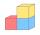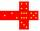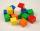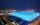# Cube root + square (second power, quadratic) - math problems

#### Number of problems found: 47

• Cube surface and volumeFind the surface of the cube with a volume of 27 dm3.
• Pyramid cutWe cut the regular square pyramid with a parallel plane to the two parts (see figure). The volume of the smaller pyramid is 20% of the volume of the original one. The bottom of the base of the smaller pyramid has a content of 10 cm2. Find the area of the
• CalculateCalculate the surface of the cube if its volume is 64 cm ^ 3.
• CalculateCalculate the length of the wall diagonal of the cube with an edge 5 cm long.
• Body diagonalThe cuboid has a volume of 32 cm3. Its side surface area is double as one of the square bases. What is the length of the body diagonal?
• Cube and sphereCube with the surface area 150 cm2 is described sphere. What is sphere surface?
• Rectangle poolFind dimensions of an open pool with a square bottom with a capacity of 32 m3 to have painted/bricked walls with the least amount of material.
• Cube edgeDetermine the edges of the cube when the surface is equal to 37.5 cm square.
• Volume and areaWhat is the volume of a cube which has an area of 361 cm2?
• The cubeThe cube has a surface area of 486 m ^ 2. Calculate its volume.
• The cubeThe surface of the cube is 150 square centimeters. Calculate: a- the content of its walls b - the length of its edges c - its volume
• Cube - wallV kocke ABCDEFGH je |CF|=4 √ 2. Aký je povrch kocky?
• Cube 6The surface area of one wall cube is 1600 cm square. How many liters of water can fit into the cube?
• Cube 6Volume of the cube is 216 cm3, calculate its surface area.
• Cube 8The surface of the cube is 0.54 m2. Calculate the length of the cube edge.
• Chemical parisonThe blown parison (with shape of a sphere) have a volume 1.5 liters. What is its surface?
• Prism XThe prism with the edges of the lengths x cm, 2x cm, and 3x cm has volume 20250 cm3. What is the area of the surface of the prism?
• BallsThree metal balls with volumes V1=71 cm3 V2=78 cm3 and V3=64 cm3 melted into one ball. Determine it's surface area.
• Rotary coneRotary cone whose height is equal to the circumference of the base, has a volume 229 cm3. Calculate the radius of the base circle and height of the cone.
• Cube in a sphereThe cube is inscribed in a sphere with a volume 7253 cm3. Determine the length of the edges of a cube.

Do you have an exciting math question or word problem that you can't solve? Ask a question or post a math problem, and we can try to solve it.

We will send a solution to your e-mail address. Solved examples are also published here. Please enter the e-mail correctly and check whether you don't have a full mailbox.

Cube root - math problems. Square (second power, quadratic) - math problems.